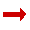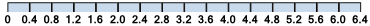# Dividing Decimals

The trick is to get rid of the decimal point from the number we are dividing by.

How? We can "shift the decimal point" out of the way by multiplying by 10, as many times as we need to.

But we must do the same thing to both numbers in the division.

### Example: 15 divided by 0.2

Let us multiply the 0.2 by 10, which shifts the decimal point out of the way:

0.2 × 10 = 2

But we must also do it to the 15:

15 × 10 = 150

So 15 ÷ 0.2 has become 150 ÷ 2 (they are both 10 times larger):

150 ÷ 2 = 75

15 ÷ 0.2 = 75The number we divide by is called the divisor.

To divide decimal numbers:

Multiply the divisor by as many 10's as necessary until we get a whole number.
Remember to multiply the dividend by the same number of 10's.

To multiply by 10 it is easier to just "shift the decimal":

### Example: Divide 6.4 by 0.4

Let us just shift the decimal point one space for both:

 move 1 6.464 0.44 move 1

6.4/0.4 is exactly the same as 64/4,
as we moved the decimal point of both numbers.

Now we can calculate:

64 / 4 = 16

6.4 / 0.4 = 16

Are there really 16 lots of 0.4 in 6.4? Let's see:For harder questions we may need to use Long Division:

### Example: Divide 0.539 by 0.11

Move the decimal point so the divisor (0.11) is a whole number:

 move 2 spaces 0.5395.3953.9 0.111.111 move 2 spaces

But what about 53.9? It still has a decimal point.

Well, we can ignore the decimal point in the dividend so long as we remember to put it back later. First we do the calculation without the decimal point:

 049 11)539      0      53      44       99       99        0

Now put the decimal point in the answer directly above the decimal point in the dividend:

 04.9 11)53.9

Another example:

### Example: Divide 9.1 by 7

We don't need to shift the decimal point at all, as the divisor (7) is already a whole number.

Ignore the decimal point in the dividend and use Long Division:

 13 7)91    7    21    21     0

Put the decimal point in the answer directly above the decimal point in the dividend:

 1.3 7)9.1